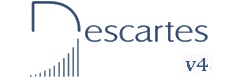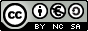Fifth and sixth year of Primary  Education INDEX Introduction 2- The properties of addition 3- Magic square of 3x3 4- Magic square of 4x4 5- 3x3 square plus number 6- 4x4 square plus number 7- 3x3 plus 3x3 squares 8- 4x4 plus 4x4 squares 9- 4x4 square formula 10- three-pointed star 11- five-pointed star 12- seven-pointed star 1- Terms of the subtraction 2- The properties of subtraction 3- Subtracting with numbers 4- 3x3 square minus number 5- 4x4 square minus number 6- 3x3 minus 3x3 squares

Operations with natural numbers I

INTRODUCTION

In this unit, basic concepts of natural numbers are revised and the operations of addition and subtraction are practised.

Numbers that appear in each window are generated at random. This allows you to use the window indefinitely and practise a lot of different exercises. Numbers consist of only one or two figures so the calculations can easily be done mentally.

Magic squares are used in the exercises, however the main objective of this unit is to learn things about natural numbers.

OBJECTIVES

• To identify values which are natural numbers
• To be able to produce a graphical representation of several natural numbers on a line.
• To put in order the values of several natural numbers
• To add up natural numbers
• To make proper use of the addition properties.
• To subtract natural numbers
• To understand how subtraction is different from addition. The position of the numbers has to be preserved and the larger one has to be put first.
• To train in mental calculation of addition and subtraction
 Eduardo Barbero CorralSpanish Ministry of Education. Year 2007